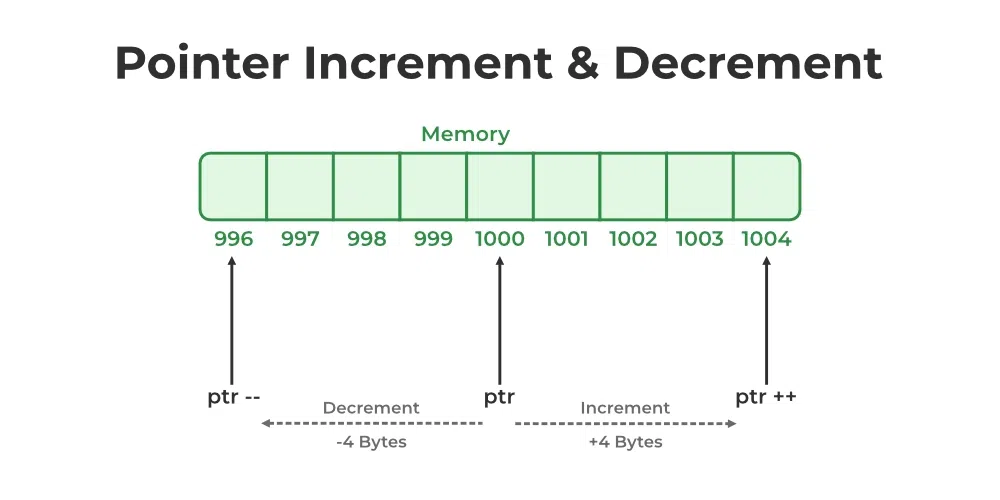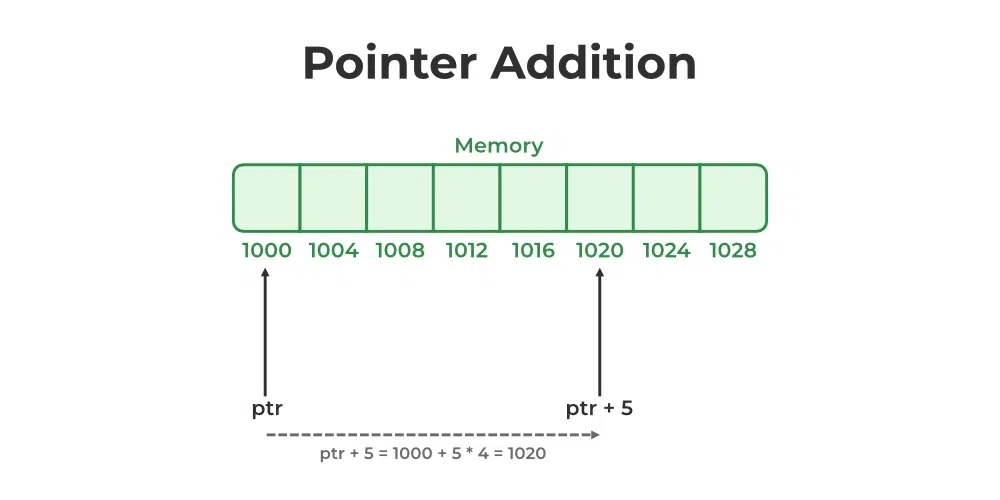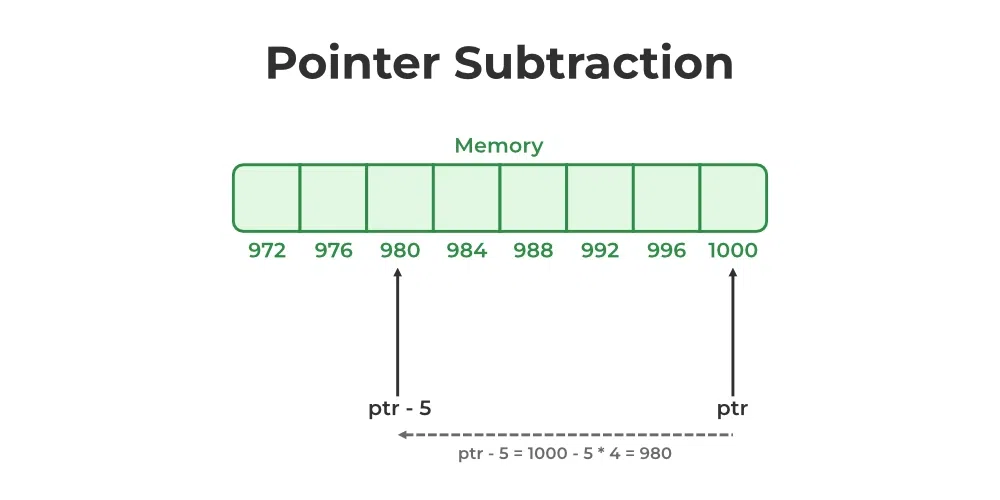GeeksforGeeks App
Open AppBrowser
Continue

# Pointer Arithmetics in C with Examples

Pointer Arithmetic is the set of valid arithmetic operations that can be performed on pointers. The pointer variables store the memory address of another variable. It doesn’t store any value.

Hence, there are only a few operations that are allowed to perform on Pointers in C language. The C pointer arithmetic operations are slightly different from the ones that we generally use for mathematical calculations. These operations are:

1. Increment/Decrement of a Pointer
2. Addition of integer to a pointer
3. Subtraction of integer to a pointer
4. Subtracting two pointers of the same type
5. Comparison of pointers of the same type

## 1. Increment/Decrement of a Pointer

Increment: It is a condition that also comes under addition. When a pointer is incremented, it actually increments by the number equal to the size of the data type for which it is a pointer.

For Example:
If an integer pointer that stores address 1000 is incremented, then it will increment by 4(size of an int), and the new address will point to 1004. While if a float type pointer is incremented then it will increment by 4(size of a float) and the new address will be 1004.

Decrement: It is a condition that also comes under subtraction. When a pointer is decremented, it actually decrements by the number equal to the size of the data type for which it is a pointer.

For Example:
If an integer pointer that stores address 1000 is decremented, then it will decrement by 4(size of an int), and the new address will point to 996. While if a float type pointer is decremented then it will decrement by 4(size of a float) and the new address will be 996.Note: It is assumed here that the architecture is 64-bit and all the data types are sized accordingly. For example, integer is of 4 bytes.

### Example of Pointer Increment and Decrement

Below is the program to illustrate pointer increment/decrement:

## C

 `#include ``// pointer increment and decrement``//pointers are incremented and decremented by the size of the data type they point to ``int` `main()``{``    ``int` `a = 22;``    ``int` `*p = &a;``    ``printf``(``"p = %u\n"``, p); ``// p = 6422288``    ``p++;``    ``printf``(``"p++ = %u\n"``, p); ``//p++ = 6422292    +4   // 4 bytes``    ``p--;``    ``printf``(``"p-- = %u\n"``, p); ``//p-- = 6422288     -4   // restored to original value`` ` `    ``float` `b = 22.22;``    ``float` `*q = &b;``    ``printf``(``"q = %u\n"``, q);  ``//q = 6422284``    ``q++;``    ``printf``(``"q++ = %u\n"``, q); ``//q++ = 6422288      +4   // 4 bytes``    ``q--;``    ``printf``(``"q-- = %u\n"``, q); ``//q-- = 6422284       -4  // restored to original value`` ` `    ``char` `c = ``'a'``;``    ``char` `*r = &c;``    ``printf``(``"r = %u\n"``, r);   ``//r = 6422283``    ``r++;``    ``printf``(``"r++ = %u\n"``, r);   ``//r++ = 6422284     +1   // 1 byte``    ``r--;``    ``printf``(``"r-- = %u\n"``, r);   ``//r-- = 6422283     -1  // restored to original value`` ` `    ``return` `0;``}`

Output

```p = 1441900792
p++ = 1441900796
p-- = 1441900792
q = 1441900796
q++ = 1441900800
q-- = 1441900796
r = 1441900791
r++ = 1441900792
r-- = 1441900791```

Note: Pointers can be outputted using %p, since, most of the computers store the address value in hexadecimal form using %p gives the value in that form. But for simplicity and understanding we can also use %u to get the value in Unsigned int form.

## 2. Addition of Integer to Pointer

When a pointer is added with an integer value, the value is first multiplied by the size of the data type and then added to the pointer.

For Example:
Consider the same example as above where the ptr is an integer pointer that stores 1000 as an address. If we add integer 5 to it using the expression, ptr = ptr + 5, then, the final address stored in the ptr will be ptr = 1000 + sizeof(int) * 5 = 1020.## C

 `// C program to illustrate pointer Addition``#include `` ` `// Driver Code``int` `main()``{``    ``// Integer variable``    ``int` `N = 4;`` ` `    ``// Pointer to an integer``    ``int` `*ptr1, *ptr2;`` ` `    ``// Pointer stores the address of N``    ``ptr1 = &N;``    ``ptr2 = &N;`` ` `    ``printf``(``"Pointer ptr2 before Addition: "``);``    ``printf``(``"%p \n"``, ptr2);`` ` `    ``// Addition of 3 to ptr2``    ``ptr2 = ptr2 + 3;``    ``printf``(``"Pointer ptr2 after Addition: "``);``    ``printf``(``"%p \n"``, ptr2);`` ` `    ``return` `0;``}`

Output

```Pointer ptr2 before Addition: 0x7ffca373da9c
Pointer ptr2 after Addition: 0x7ffca373daa8 ```

## 3. Subtraction  of Integer to Pointer

When a pointer is subtracted with an integer value, the value is first multiplied by the size of the data type and then subtracted from the pointer similar to an addition.

For Example:
Consider the same example as above where the ptr is an integer pointer that stores 1000 as an address. If we subtract integer 5 from it using the expression, ptr = ptr – 5, then, the final address stored in the ptr will be ptr = 1000 – sizeof(int) * 5 = 980.### Example of Subtraction of Integer from Pointer

Below is the program to illustrate pointer Subtraction:

## C

 `// C program to illustrate pointer Subtraction``#include `` ` `// Driver Code``int` `main()``{``    ``// Integer variable``    ``int` `N = 4;`` ` `    ``// Pointer to an integer``    ``int` `*ptr1, *ptr2;`` ` `    ``// Pointer stores the address of N``    ``ptr1 = &N;``    ``ptr2 = &N;`` ` `    ``printf``(``"Pointer ptr2 before Subtraction: "``);``    ``printf``(``"%p \n"``, ptr2);`` ` `    ``// Subtraction of 3 to ptr2``    ``ptr2 = ptr2 - 3;``    ``printf``(``"Pointer ptr2 after Subtraction: "``);``    ``printf``(``"%p \n"``, ptr2);`` ` `    ``return` `0;``}`

Output

```Pointer ptr2 before Subtraction: 0x7ffd718ffebc
Pointer ptr2 after Subtraction: 0x7ffd718ffeb0 ```

## 4. Subtraction of Two Pointers

The subtraction of two pointers is possible only when they have the same data type. The result is generated by calculating the difference between the addresses of the two pointers and calculating how many bits of data it is according to the pointer data type. The subtraction of two pointers gives the increments between the two pointers.

For Example:
Two integer pointers say ptr1(address:1000) and ptr2(address:1004) are subtracted. The difference between addresses is 4 bytes. Since the size of int is 4 bytes, therefore the increment between ptr1 and ptr2 is given by (4/4) = 1.

### Example of Subtraction of Two Pointer

Below is the implementation to illustrate the Subtraction of Two Pointers:

## C

 `// C program to illustrate Subtraction``// of two pointers``#include `` ` `// Driver Code``int` `main()``{``    ``int` `x = 6; ``// Integer variable declaration``    ``int` `N = 4;`` ` `    ``// Pointer declaration``    ``int` `*ptr1, *ptr2;`` ` `    ``ptr1 = &N; ``// stores address of N``    ``ptr2 = &x; ``// stores address of x`` ` `    ``printf``(``" ptr1 = %u, ptr2 = %u\n"``, ptr1, ptr2);``    ``// %p gives an hexa-decimal value,``    ``// We convert it into an unsigned int value by using %u`` ` `    ``// Subtraction of ptr2 and ptr1``    ``x = ptr1 - ptr2;`` ` `    ``// Print x to get the Increment``    ``// between ptr1 and ptr2``    ``printf``(``"Subtraction of ptr1 "``           ``"& ptr2 is %d\n"``,``           ``x);`` ` `    ``return` `0;``}`

Output

``` ptr1 = 2715594428, ptr2 = 2715594424
Subtraction of ptr1 & ptr2 is 1```

## 5. Comparison of pointers of the same type

We can compare the two pointers by using the comparison operators in C. We can implement this by using all operators in C >, >=, <, <=, ==, !=.  It returns true for the valid condition and returns false for the unsatisfied condition.

1. Step 1: Initialize the integer values and point these integer values to the pointer.
2. Step 2: Now, check the condition by using comparison or relational operators on pointer variables.
3. Step 3: Display the output.

## C

 `// C Program to illustrate the pointer comparison``#include `` ` `int` `main()``{`` ` `    ``// code``    ``int` `num1 = 5, num2 = 6, num3 = 5; ``// integer input``    ``int``* p1``        ``= &num1; ``// addressing the integer input to pointer``    ``int``* p2 = &num2;``    ``int``* p3 = &num3;``    ``// comparing the pointer variables.``    ``if` `(*p1 < *p2) {``        ``printf``(``"\n%d less than %d"``, *p1, *p2);``    ``}``    ``if` `(*p2 > *p1) {``        ``printf``(``"\n%d greater than %d"``, *p2, *p1);``    ``}``    ``if` `(*p3 == *p1) {``        ``printf``(``"\nBoth the values are equal"``);``    ``}``    ``if` `(*p3 != *p2) {``        ``printf``(``"\nBoth the values are not equal"``);``    ``}`` ` `    ``return` `0;``}`

Output

```5 less than 6
6 greater than 5
Both the values are equal
Both the values are not equal```

### Comparison to NULL

A pointer can be compared or assigned a NULL value irrespective of what is the pointer type. Such pointers are called NULL pointers and are used in various pointer-related error-handling methods.

## C

 `// C Program to demonstrate the pointer comparison with NULL``// value``#include `` ` `int` `main()``{`` ` `    ``int``* ptr = NULL;`` ` `    ``if` `(ptr == NULL) {``        ``printf``(``"The pointer is NULL"``);``    ``}``    ``else` `{``        ``printf``(``"The pointer is not NULL"``);``    ``}``    ``return` `0;``}`

Output

`The pointer is NULL`

### Comparison operators on Pointers using an array

In the below approach, it results in the count of odd numbers and even numbers in an array. We are going to implement this by using a pointer.

1. Step 1: First, declare the length of an array and array elements.
2. Step 2: Declare the pointer variable and point it to the first element of an array.
3. Step 3: Initialize the count_even and count_odd. Iterate the for loop and check the conditions for the number of odd elements and even elements in an array
4. Step 4: Increment the pointer location ptr++ to the next element in an array for further iteration.
5. Step 5: Print the result.

## C

 `#include `` ` `int` `main()``{``    ``int` `n = 10; ``// length of an array`` ` `    ``int` `arr[] = { 1, 2, 3, 4, 5, 6, 7, 8, 9, 10 };``    ``int``* ptr; ``// Declaration of pointer variable`` ` `    ``ptr = arr; ``// Pointer points the first (0th index)``               ``// element in an array``    ``int` `count_even = 0;``    ``int` `count_odd = 0;`` ` `    ``for` `(``int` `i = 0; i < n; i++) {`` ` `        ``if` `(*ptr % 2 == 0) {``            ``count_even++;``        ``}``        ``if` `(*ptr % 2 != 0) {``            ``count_odd++;``        ``}``        ``ptr++; ``// Pointing to the next element in an array``    ``}``    ``printf``(``"\n No of even elements in an array is : %d"``,``           ``count_even);``    ``printf``(``"\n No of odd elements in an array is : %d"``,``           ``count_odd);``}`

Output

``` No of even elements in an array is : 5
No of odd elements in an array is : 5```

## Pointer Arithmetic on Arrays

Pointers contain addresses. Adding two addresses makes no sense because there is no idea what it would point to. Subtracting two addresses lets you compute the offset between the two addresses. An array name acts like a pointer constant. The value of this pointer constant is the address of the first element.

For Example: if an array is named arr then arr and &arr can be used to reference the array as a pointer.

Below is the program to illustrate the Pointer Arithmetic on arrays:

Program 1:

## C

 `// C program to illustrate the array``// traversal using pointers``#include `` ` `// Driver Code``int` `main()``{`` ` `    ``int` `N = 5;`` ` `    ``// An array``    ``int` `arr[] = { 1, 2, 3, 4, 5 };`` ` `    ``// Declare pointer variable``    ``int``* ptr;`` ` `    ``// Point the pointer to first``    ``// element in array arr[]``    ``ptr = arr;`` ` `    ``// Traverse array using ptr``    ``for` `(``int` `i = 0; i < N; i++) {`` ` `        ``// Print element at which``        ``// ptr points``        ``printf``(``"%d "``, ptr);``        ``ptr++;``    ``}``}`

Output

`1 2 3 4 5 `

Program 2:

## C

 `// C program to illustrate the array``// traversal using pointers in 2D array``#include `` ` `// Function to traverse 2D array``// using pointers``void` `traverseArr(``int``* arr, ``int` `N, ``int` `M)``{`` ` `    ``int` `i, j;`` ` `    ``// Traverse rows of 2D matrix``    ``for` `(i = 0; i < N; i++) {`` ` `        ``// Traverse columns of 2D matrix``        ``for` `(j = 0; j < M; j++) {`` ` `            ``// Print the element``            ``printf``(``"%d "``, *((arr + i * M) + j));``        ``}``        ``printf``(``"\n"``);``    ``}``}`` ` `// Driver Code``int` `main()``{`` ` `    ``int` `N = 3, M = 2;`` ` `    ``// A 2D array``    ``int` `arr[] = { { 1, 2 }, { 3, 4 }, { 5, 6 } };`` ` `    ``// Function Call``    ``traverseArr((``int``*)arr, N, M);``    ``return` `0;``}`

Output

```1 2
3 4
5 6 ```

My Personal Notes arrow_drop_up